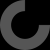# Report as inappropriate

Nice project!

There is an issue however they way you do chamfers, because at resolution 1 the cylinder degenerates into a prism, but keeps the same orientation during the minkowski().

This has 2 consequences:

• the boxes are smaller than the requested dimensions as the base / height of the prism are smaller than the diameter of the cylinder
• the panels are only chamfered at the bottom (and are too short as well)

(It becomes very apparent when you use bigger chamfers)

```It is less elegant, but making "real" chamfer in RoundBox gives better results:
module RoundBox(\$a=Length, \$b=Width, \$c=Height){// Cube bords arrondis
if (Resolution == 1) {
f = sqrt(2FiletFilet);
difference(){
cube ([\$a,\$b,\$c], center = false);
for (y = [0,\$b])
for (z=[0,\$c]){
translate([-0.1,y,z-Filet])
rotate([45,0,0])
cube([\$a+0.2,f,f]);
}
}
} else {
\$fn=Resolution;
translate([0,Filet,Filet]){
minkowski (){
cube ([\$a-(Length/2),\$b-(2Filet),\$c-(2Filet)], center = false);
rotate([0,90,0]){
cylinder(r=Filet,h=Length/2, center = false);
}
}
}
}
}// End of RoundBox Module